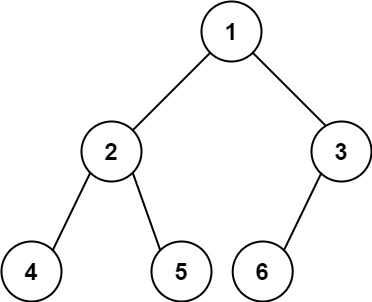304 North Cardinal St.
Dorchester Center, MA 02124

# Count Complete Tree Nodes LeetCode Solution

## Problem – Count Complete Tree Nodes LeetCode Solution

Given the `root` of a complete binary tree, return the number of the nodes in the tree.

According to Wikipedia, every level, except possibly the last, is completely filled in a complete binary tree, and all nodes in the last level are as far left as possible. It can have between `1` and `2h` nodes inclusive at the last level `h`.

Design an algorithm that runs in less than `O(n)` time complexity.

Example 1:``````Input: root = [1,2,3,4,5,6]
Output: 6
``````

Example 2:

``````Input: root = []
Output: 0
``````

Example 3:

``````Input: root = 
Output: 1
``````

Constraints:

• The number of nodes in the tree is in the range `[0, 5 * 104]`.
• `0 <= Node.val <= 5 * 104`
• The tree is guaranteed to be complete.

## Count Complete Tree Nodes LeetCode Solution in Java

``````class Solution {
int height(TreeNode root) {
return root == null ? -1 : 1 + height(root.left);
}
public int countNodes(TreeNode root) {
int h = height(root);
return h < 0 ? 0 :
height(root.right) == h-1 ? (1 << h) + countNodes(root.right)
: (1 << h-1) + countNodes(root.left);
}
}
``````

## Count Complete Tree Nodes LeetCode Solution in C++

``````class Solution {

public:

int countNodes(TreeNode* root) {

if(!root) return 0;

int hl=0, hr=0;

TreeNode *l=root, *r=root;

while(l) {hl++;l=l->left;}

while(r) {hr++;r=r->right;}

if(hl==hr) return pow(2,hl)-1;

return 1+countNodes(root->left)+countNodes(root->right);

}

};
``````

## Count Complete Tree Nodes LeetCode Solution in Python

`````` class Solution:
# @param {TreeNode} root
# @return {integer}
def countNodes(self, root):
if not root:
return 0
leftDepth = self.getDepth(root.left)
rightDepth = self.getDepth(root.right)
if leftDepth == rightDepth:
return pow(2, leftDepth) + self.countNodes(root.right)
else:
return pow(2, rightDepth) + self.countNodes(root.left)

def getDepth(self, root):
if not root:
return 0
return 1 + self.getDepth(root.left)
``````
##### Count Complete Tree Nodes LeetCode Solution Review:

In our experience, we suggest you solve this Count Complete Tree Nodes LeetCode Solution and gain some new skills from Professionals completely free and we assure you will be worth it.

If you are stuck anywhere between any coding problem, just visit Queslers to get the Count Complete Tree Nodes LeetCode Solution

Find on Leetcode

##### Conclusion:

I hope this Count Complete Tree Nodes LeetCode Solution would be useful for you to learn something new from this problem. If it helped you then don’t forget to bookmark our site for more Coding Solutions.

This Problem is intended for audiences of all experiences who are interested in learning about Data Science in a business context; there are no prerequisites.

Keep Learning!

More Coding Solutions >>

LeetCode Solutions

Hacker Rank Solutions

CodeChef Solutions